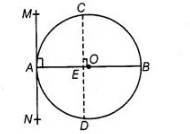# Prove that a diameter AB of a circle

Question:

Prove that a diameter AB of a circle bisects all those chords which are parallel to the tangent at the point A.

Solution:

Given, AB is a diameter of the circle.

A tangent is drawn from point A. Draw a chord CD parallel to the tangent MAN.So. $C D$ is a chord of the circle and $O A$ is a radius of the circle.

$\angle M A O=90^{\circ}$

[tanment at anv noint of a circle is perpendicular to the radius through the point of contact]

$\angle C E O=\angle M A O \quad$ [corresponding angles]

$\therefore$ $\angle C E O=90^{\circ}$

Thus, OE bisects CD, [perpendicular from centre of circle to chord bisects the chord] Similarly, the diameter AB bisects all. Chords which are

parallel to the tangent at the point A.